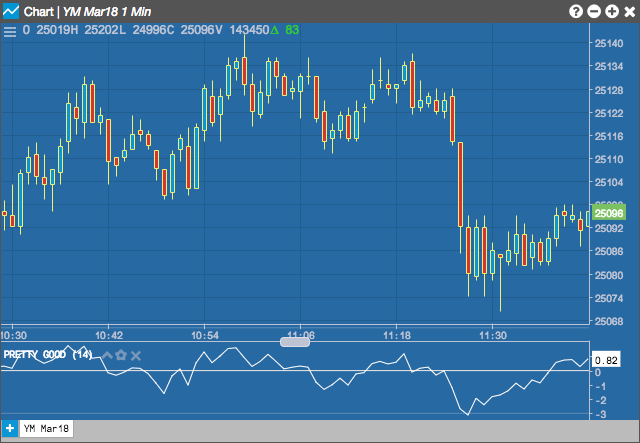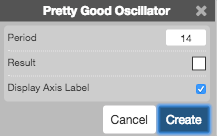Charts

# Pretty Good Oscillator

The Pretty Good Oscillator indicator by Mark Johnson measures the distance of the current close from its N-day simple moving average, expressed in terms of an average true range over a similar period.## Configuration Options• Period: Number of bars to use in the calculations.
• Color Selectors: Colors to use for graph elements.
• Display Axis Label: Whether to display the most recent value on the Y axis.

## Formula

$PGO = \frac{close - SMA[N]\ of\ closes}{EMA[N]\ of\ true\ range}$